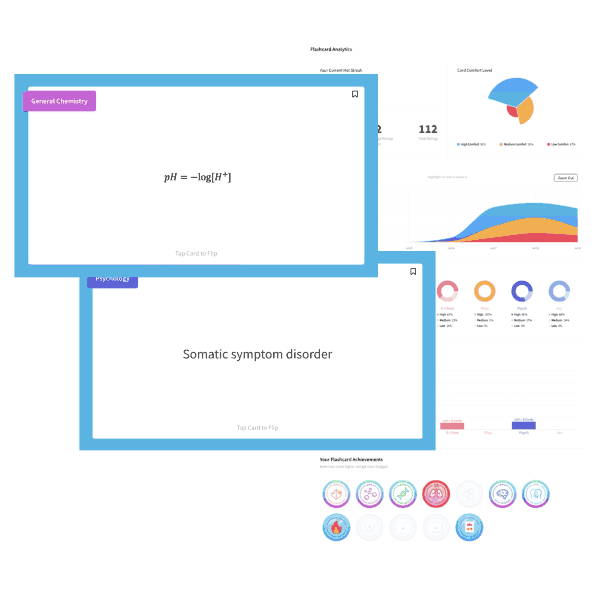# MCAT Physics – Light Behavior

• by
• Mar 17, 2015
• MCAT Blog, MCAT Physics, MCAT Question of the Day

A ray of light traveling through air hits a pane of glass with known refractive index. What information is required in order to calculate the angle of refraction?

a) Planck’s constant and the speed of light

b) the specific gravity of the glass

c) the angle of incidence

d) the density of air and the density of glass

Explanation

Snell’s law is written as η1sinθ1 =  η2sinθ2, where the refractive index of air, η1, equals 1. Given the refractive index of glass, η2, only the angle of incidence is required to calculate the angle of refraction.

a) Planck’s constant and the speed of light, incorrect, E=hf describes energy.

b) the specific gravity of the glass, incorrect, The specific gravity provides neither the angle of incidence nor the refractive index.

c) the angle of incidence, correct.

d) the density of air and the density of glass, incorrect, although the refractive index of a medium is related to its density, the density provides neither specific information about the refractive index nor the angle of incidence.

## Want more MCAT practice?

### We’ve got options for every schedule and learning style!

From the best online MCAT course created by top instructors with 524+ MCAT scores to the most representative full-length practice exams and private tutoring, we can custom tailor your MCAT prep to your goals!

Not sure which option is right for you? Schedule a free MCAT consultation with an MCAT expert using the form below. No obligation, just expert advice.

Schedule My Free Consultation